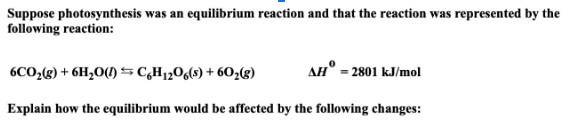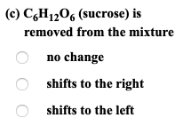# Suppose photosynthesis was an equilibrium reaction and that the reaction was represented by the following reaction: 6CO2(g) + 6H2O(l) ⇌ C6H12O6(s) + 6O2(g) ΔH° = 2801 kJ/mol Explain how the equilibrium would be affected by the following changes: (c) C6H12O6 (sucrose) is removed from the mixture a) no change b)shifts to the right c) shifts to the left